# Scattering Theory - An Overview

## by Tilo Arens

Scattering theory is concerned with the effect obstacles or inhomogeneities have on an incident waves. There are two types of problems in this area:

• The Direct Problem: This problem is concerned with determining the scattered field from the knowledge of the incident field and the scattering obstacle.
• The Inverse Problem: Here one tries to determine the shape and/or physical properties of the scatterer from the measurement of the scattered field for a number of incident fields.

The propagation of waves in a homogeneous, isotropic medium is mathematically described by the wave equation,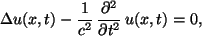where c denotes the speed of propagation. In the Brunel Rough Surface Scattering group, we are concerned with scattering problems involving time harmonic waves, i.e. wave fields of the form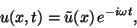where w denotes the circular frequency. Such wave fields are important for many applications in acoustics, electro-magnetic or elasticity theory. The wave equation can then be reducted to the Helmholtz equation,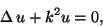where k = w/ c.

## Direct Scattering Problems

### Scattering by a bounded obstacle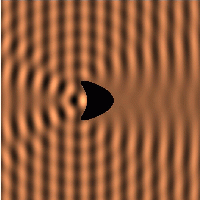The total field is usually represented as the sum of the incident and the scattered field. The scattering problem can then be formulated as a boundary value problem for the scattered field in the region outside the scattering obstacle, consisting of

• the Helmholtz equation,
• a boundary condition on the boundary of the scattering obstacle,
As the boundary condition either a Dirichlet, Neumann or Robin boundary condition is possible. In acoustics, these correspond to sound soft, sound hard or impedance surfaces of the obstacle. Sommerfeld's raditation condition is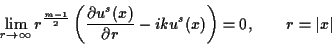uniformly in all directions x/r, where us denotes the scattered field and m = 2,3 the spacial dimension of the problem.

The image above is a visualisation of the scattered field for such a bounded obstacle problem. A plane wave is incident from the left-hand side and scattered by the kite-shaped object. A Dirichlet boundary condition (i.e. the total field vanishes) was used on the surface. For more detail on this problem, there is a separate page including an animation (Java applet) of the wave field.

### Scattering by a diffraction gratingA diffraction grating is a very simple type of unbounded scatterer: It is a surface that is invariant in the, say x3 coordinate direction while showing some periodicity in the x1 direction. If the incident field is also constant in the x3 direction, the scattering problem can be treated as a 2-dimensional problem. The diffraction grating can then be described as the graph of a function f that is periodic with, say, period L.

If the incident field also is periodic with a certain, possibly different, period (which is the case for an incident plane wave!), both the incident and the scattered field will be quasi-periodic. This property allows substantial simplifications as the problem can thus be dealt with in spaces of periodic functions.

A further difficulty arises, in that the scattered field in a diffraction problem will, in general, not show any decay in directions parallel to the surface. Thus, Sommerfeld's radiation condition will not apply. It is replaced by the Rayleigh Expansion Radiation Condition (RERC), that the scattered field has, at some height above the surface, an expansion in plane waves propagating upwards and evanescent waves, decaying exponentially with distance from the surface.

Numerically, the problem can be treated, for example, by a boundary integral ansatz with a Nyström method for the numerical treatment of the resulting boundary integral equation. This type of method is extremely efficient, and a code developed by the Brunel Rough Surface Scattering group clearly demonstrates the theoretically expected exponential convergence rates. There is also a more detailed description of the problem available, that includes an animation of the total field based on a Java applet.

### Scattering by a rough surface

Of special interest to the Brunel Rough Surface Scattering group are problems involving effectively unbounded obstacles. Such an obstacle is termed a rough surface, if it can be represented as the graph of a bounded, continuous function f. Often, f will be required to satisfy some additional smoothness properties, usually Lipschitz continuity of its first derivative.

A number of difficulties arise when dealing with such problems. Firstly, to formulate the problem as a boundary value problem, a suitable radiation condition has to be imposed. Such a condition is the upward propagating radiation condition introduced by Chandler-Wilde. It was shown that this radiation condition ensures uniqueness of solution to the sound soft and impedance surface scattering problems for quite general classes of incident fields, including cylindrical and plane waves.

Secondly, when deriving boundary integral equation formulations, the standard fundamental solution, i.e. the Green's function in free field conditions, turns out to be poor choice as the kernel in single- and double-layer potentials. This is due to the fact that we cannot expect the surface density to be decaying along the infinite surface for general classes of incident waves. Thus, the decay rate of the free field Green's function, i.e.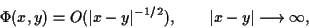is not enough to ensure existence of the boundary integral for surface densities that are merely bounded. Thus one has to turn to alternatives, for example the Greens function G(x,y) for a half plane with Dirichlet boundary conditions. This function shows the decay rate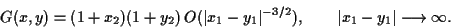Thus, for a scattering surface f, bounded in x2-direction, the boundary integrals exist, even if the density is merely bounded.

However, and this is a further difficulty, the arising integral operators have an unbounded range of integration. Even for the simplest case, a convolution operator, one observes that such an operator is not compact. Thus, an entirely new solvability theory is required to prove that the boundary integral equation admits a unique solution.

The extension of these results to the full, 3-dimensional case, is the object of much ongoing research in the Brunel Rough Surface Scattering group.

### Elastic Wave Scattering

If one considers the propagation of waves in an elastic solid, very similar types of scattering problems occur. However, even in the simplest case of an isotropic, homogeneous medium of propagation, the elastic wave motion is much more complicated than in the acoustic or electro-magnetic case. This is due to the fact that there are two types of waves, compressional (P) and shear (S) waves, that propagate through the medium at different wave speeds cp and cs, respectively. These two wave types are coupled through the boundary conditions: even a plane P-wave incident onto a planar surface will give rise to both a reflected P- and S-wave, with different directions of propagation. In an isotropic, homogeneous medium, the wave motion of time-harmonic fields is described by the Navier equation,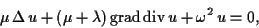where m, l (m > 0, m + l > 0) denote the Lame constants and and w > 0 the circular frequency. This equation can be considered in either two or three dimensions. The investigation of the above three types of scattering problems is also possible via boundary integral equation methods. For the bounded obstacle case, these methods are well-known, while the first proofs of well-posedness of the boundary integral equation formulations for diffraction grating problems or rough surfaces can be found in some of the author's papers.

## Inverse Scattering Problems

Inverse problems are problems of reconstruction of parameters in a mathematical problems from (partial) knowledge of the problems solution. An examples is the reconstruction of coefficients in a PDE from knowledge of the Cauchy Data of the solution on the boundary of the domain. Such problems are allmost always non-linear and ill-posed. Thus they require regularisation methods for their solution.

### Reconstruction of a bounded obstacle

An inverse scattering problem consists in the reconstruction of a scattering obstacle from the knowledge of the far-field data for one or a number of incident fields. There are currently three methods available for the solution of such a problem:
• iterative methods relying on a Newton type iteration,
• methods splitting up the problem in the ill-posed linear problem of reconstructing the scattered field from the far-field pattern and the well-posed non-linear problem of finding the boundary from the scattered field,
• methods using a direct characterisation of the obstacle.

## References

### Books

• D. Colton and R. Kress, Integral Equation Methods in Scattering Theory, Wiley, 1983.
• D. Colton and R. Kress, Inverse Acoustic and Electromagnetic Scattering Theory, 2nd edition, Springer-Verlag, 1998.
• A. Kirsch, An Introduction to the Mathematical Theory of Inverse Problems, Springer-Verlag, 1996.

### Papers by members of the Brunel group

The following members of the Brunel Rough Surface Scattering group maintain a list of their publications on separate pages:

### Other Resources

• There are some nice examples of scattering problems and related numerical methods on the home page of Klaus Giebermann at Bonn University.

Page written and maintained by Tilo Arens
last change: 5 January 2001
Mathematical formulae by latex2html